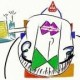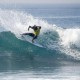Skip to content

This is the 9th post in a series attempting to recreate the figures in Lattice: Multivariate Data Visualization with R (R code available here) with ggplot2.

Previous parts in this series: Part 1, Part 2, Part 3, Part 4, Part 5, Part 6, Part 7, Part 8.

## Chapter 9 – Labels and Legends

Topics covered:

• Labels (main, sub, xlab, ylab)
• Legends, color keys
• Legends in grouped displays; auto.key
• Dropping unused levels of grouping variable
• Page annotation

### Figure 9.1

 ```> library(lattice) > library(ggplot2)```
 `> data(Cars93, package = "MASS")`

lattice

 ```> sup.sym <- Rows(trellis.par.get("superpose.symbol"), + 1:5)```
 ```> pl <- xyplot(Price ~ EngineSize | reorder(AirBags, Price), + data = Cars93, groups = Cylinders, subset = Cylinders != + "rotary", scales = list(y = list(log = 2, tick.number = 3)), + xlab = "Engine Size (litres)", ylab = "Average Price (1000 USD)", + key = list(text = list(levels(Cars93\$Cylinders)[1:5]), + points = sup.sym, space = "right")) > print(pl)```

ggplot2

 `> Cars93\$AirBags <- with(Cars93, reorder(AirBags, Price))`
 ```> pg <- ggplot(Cars93, aes(EngineSize, Price, colour = factor(Cylinders))) + + geom_point(subset = .(Cylinders != "rotary")) + facet_grid(~AirBags) + + xlab("Engine Size (litres)") + ylab("Average Price (1000 USD)") + + scale_y_log2() > print(pg)```

### Figure 9.2

lattice

 ```> my.pch <- c(21:25, 20) > my.fill <- c("transparent", "grey", "black")```
 ```> pl <- with(Cars93, xyplot(Price ~ EngineSize, scales = list(y = list(log = 2, + tick.number = 3)), panel = function(x, y, ..., subscripts) { + pch <- my.pch[Cylinders[subscripts]] + fill <- my.fill[AirBags[subscripts]] + panel.xyplot(x, y, pch = pch, fill = fill, col = "black") + }, key = list(space = "right", adj = 1, text = list(levels(Cylinders)), + points = list(pch = my.pch), text = list(levels(AirBags)), + points = list(pch = 21, fill = my.fill), rep = FALSE))) > print(pl)```

ggplot2

 ```> pg <- ggplot(Cars93, aes(EngineSize, Price, shape = factor(Cylinders), + colour = factor(AirBags))) + geom_point() + scale_y_log2() > print(pg)```

### Figure 9.3

lattice

 ```> library(latticeExtra) > hc1 <- hclust(dist(USArrests, method = "canberra")) > hc1 <- as.dendrogram(hc1) > ord.hc1 <- order.dendrogram(hc1) > hc2 <- reorder(hc1, state.region[ord.hc1]) > ord.hc2 <- order.dendrogram(hc2) > region.colors <- trellis.par.get("superpose.polygon")\$col```
 ```> pl <- levelplot(t(scale(USArrests))[, ord.hc2], scales = list(x = list(rot = 90)), + colorkey = FALSE, legend = list(right = list(fun = dendrogramGrob, + args = list(x = hc2, ord = ord.hc2, side = "right", + size = 10, size.add = 0.5, add = list(rect = list(col = "transparent", + fill = region.colors[state.region])), + type = "rectangle")))) > print(pl)```

ggplot2

 ```> USArrests2 <- melt(t(scale(USArrests))) > USArrests2\$X2 <- factor(USArrests2\$X2, levels = state.name[ord.hc2])```
 ```> pg <- ggplot(USArrests2, aes(X1, X2, fill = value)) + + geom_tile() + opts(axis.text.x = theme_text(angle = 90, + hjust = 1, colour = "grey50")) + opts(aspect.ratio = 50/5) > print(pg)```
 Note ggplot2 does not support the inclusion of dendrograms in the legend.
6 Comments leave one →
1.August 10, 2009 1:39 pm

It would be nice if the ggplot legend could drop the background colour in legends. Compared with lattice the legend often looks clumpsy. But the syntax is nice I think.

•learnr permalink*
August 11, 2009 4:37 pm

I have opted to use the default settings.

Nevertheless, `opts(legend.key = theme_blank())` should drop the legend key background fill and colour.

2.March 23, 2011 8:34 pm

Is it possible to add scale to lattice cluster?
And is it possible to set scale color to white to blue ?

•learnr permalink*
March 23, 2011 9:25 pm

I am fairly certain this is possible, however I do not know much about lattice, so am not able to answer your queries.